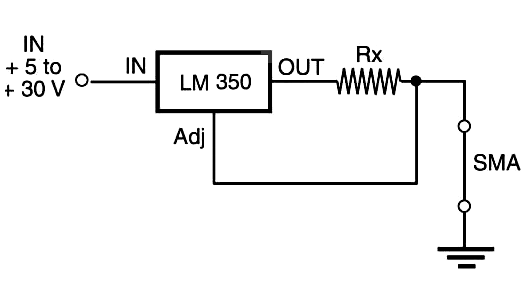## Constant Current Source (MEC144E)

This circuit described here is based on my book Robotics, Mechatronics and Artificial Intelligence (sold out) published in the United States. It can be used to control SMA (Shape Memory Alloy) and other DC loads in robotics and mechatronics projects. The resistance of a SMA changes when it is heated means that the current sourced by the power supply also changes. This variation can cause overheating or other problems. If the SMA is powered from a constant current source, secure operation can be achieved.

The block shown by Figure 1 can be used to maintain constant current across an SMA in the range from few milliamperes to 3 A. The resistor, according to the desired current, is calculated by the following formula:

## R = 1.25 / I

Where

R = resistance of R in ohm

I = desired current in the SMA

Other constant current sources described in this section can be used. In particular, we recommend MEC131E, which uses a common bipolar transistor for the task.Figure 1 Constant current source.